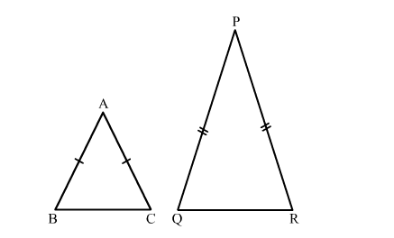# Two isosceles triangles have equal vertical angles and their areas are in the ratio 36 : 25. Find the ratio of their corresponding heights.

Question:

Two isosceles triangles have equal vertical angles and their areas are in the ratio 36 : 25. Find the ratio of their corresponding heights.

Solution:

Given: Two isosceles triangles have equal vertical angles and their areas are in the ratio of 36:25.

To find: Ratio of their corresponding heights.Suppose $\triangle \mathrm{ABC}$ and $\triangle \mathrm{PQR}$ are two isosceles triangles with $\angle \mathrm{A}=\angle \mathrm{P}$.

Now, AB = AC and PQ = PR

$\therefore \mathrm{ABAC}=\mathrm{PQPR}$

In $\triangle \mathrm{ABC}$ and $\triangle \mathrm{PQR}$,

$\angle \mathrm{A}=\angle \mathrm{P}$

$\mathrm{ABAC}=\mathrm{PQPR}$

$\therefore \triangle \mathrm{ABC} \sim \triangle \mathrm{PQR} \quad$ (SAS Similarity)

Let $A D$ and $P S$ be the altitudes of $\triangle A B C$ and $\triangle P Q R$, respectively.

We know that the ratio of areas of two similar triangles is equal to the ratio of squares of their corresponding altitudes.

$\therefore \operatorname{ar} \Delta \mathrm{ABCar} \Delta \mathrm{PQR}=\mathrm{ADPS} 2 \Rightarrow 3625=\mathrm{ADPS} 2 \Rightarrow \mathrm{ADPS}=65$

Hence, the ratio of their corresponding heights is $6: 5$.